Search by Topic

Resources tagged with Triangles similar to Triangle Transformation:

Filter by: Content type:
Age range:
Challenge level:

There are 58 results

Broad Topics > Angles, Polygons, and Geometrical Proof > TrianglesTriangle Transformation

Age 7 to 14 Challenge Level:

Start with a triangle. Can you cut it up to make a rectangle?Kissing Triangles

Age 11 to 14 Challenge Level:

Determine the total shaded area of the 'kissing triangles'.Liethagoras' Theorem

Age 7 to 14

Liethagoras, Pythagoras' cousin (!), was jealous of Pythagoras and came up with his own theorem. Read this article to find out why other mathematicians laughed at him.Squareo'scope Determines the Kind of Triangle

Age 11 to 14

A description of some experiments in which you can make discoveries about triangles.Isosceles

Age 11 to 14 Challenge Level:

Prove that a triangle with sides of length 5, 5 and 6 has the same area as a triangle with sides of length 5, 5 and 8. Find other pairs of non-congruent isosceles triangles which have equal areas.Dotty Triangles

Age 11 to 14 Challenge Level:

Imagine an infinitely large sheet of square dotty paper on which you can draw triangles of any size you wish (providing each vertex is on a dot). What areas is it/is it not possible to draw?Trice

Age 11 to 14 Challenge Level:

ABCDEFGH is a 3 by 3 by 3 cube. Point P is 1/3 along AB (that is AP : PB = 1 : 2), point Q is 1/3 along GH and point R is 1/3 along ED. What is the area of the triangle PQR?Triangular Tantaliser

Age 11 to 14 Challenge Level:

Draw all the possible distinct triangles on a 4 x 4 dotty grid. Convince me that you have all possible triangles.Ratty

Age 11 to 14 Challenge Level:

If you know the sizes of the angles marked with coloured dots in this diagram which angles can you find by calculation?Tetrahedra Tester

Age 11 to 14 Challenge Level:

An irregular tetrahedron is composed of four different triangles. Can such a tetrahedron be constructed where the side lengths are 4, 5, 6, 7, 8 and 9 units of length?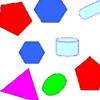Part the Polygons

Age 7 to 11 Short Challenge Level:

Draw three straight lines to separate these shapes into four groups - each group must contain one of each shape.Floored

Age 11 to 14 Challenge Level:

A floor is covered by a tessellation of equilateral triangles, each having three equal arcs inside it. What proportion of the area of the tessellation is shaded?Isosceles Triangles

Age 11 to 14 Challenge Level:

Draw some isosceles triangles with an area of $9$cm$^2$ and a vertex at (20,20). If all the vertices must have whole number coordinates, how many is it possible to draw?Cut it Out

Age 7 to 11 Challenge Level:

Can you dissect an equilateral triangle into 6 smaller ones? What number of smaller equilateral triangles is it NOT possible to dissect a larger equilateral triangle into?Counting Triangles

Age 11 to 14 Challenge Level:

Triangles are formed by joining the vertices of a skeletal cube. How many different types of triangle are there? How many triangles altogether?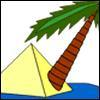Triangle Island

Age 7 to 11 Challenge Level:

You have pitched your tent (the red triangle) on an island. Can you move it to the position shown by the purple triangle making sure you obey the rules?Making Maths: Equilateral Triangle Folding

Age 7 to 14 Challenge Level:

Make an equilateral triangle by folding paper and use it to make patterns of your own.Triangle Inequality

Age 11 to 14 Challenge Level:

ABC is an equilateral triangle and P is a point in the interior of the triangle. We know that AP = 3cm and BP = 4cm. Prove that CP must be less than 10 cm.Uncanny Triangles

Age 7 to 11 Challenge Level:

Can you help the children find the two triangles which have the lengths of two sides numerically equal to their areas?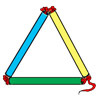Making Maths: Test the Strength of a Triangle

Age 7 to 11 Challenge Level:

Have you noticed that triangles are used in manmade structures? Perhaps there is a good reason for this? 'Test a Triangle' and see how rigid triangles are.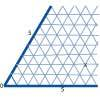Cartesian Isometric

Age 7 to 11 Challenge Level:

The graph below is an oblique coordinate system based on 60 degree angles. It was drawn on isometric paper. What kinds of triangles do these points form?Triangle Pin-down

Age 7 to 11 Challenge Level:

Use the interactivity to investigate what kinds of triangles can be drawn on peg boards with different numbers of pegs.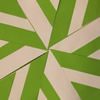Folding Flowers 2

Age 7 to 11 Challenge Level:

Make a flower design using the same shape made out of different sizes of paper.Name That Triangle!

Age 7 to 11 Challenge Level:

Can you sketch triangles that fit in the cells in this grid? Which ones are impossible? How do you know?Constructing Triangles

Age 11 to 14 Challenge Level:

Generate three random numbers to determine the side lengths of a triangle. What triangles can you draw?Inside Seven Squares

Age 7 to 11 Challenge Level:

What is the total area of the four outside triangles which are outlined in red in this arrangement of squares inside each other?Lighting up Time

Age 7 to 14 Challenge Level:

A very mathematical light - what can you see?Rectangle Tangle

Age 7 to 11 Challenge Level:

The large rectangle is divided into a series of smaller quadrilaterals and triangles. Can you untangle what fractional part is represented by each of the shapes?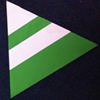Regular Rings 1

Age 7 to 11 Challenge Level:

Can you work out what shape is made by folding in this way? Why not create some patterns using this shape but in different sizes?Cut and Make

Age 7 to 11 Challenge Level:

Cut a square of paper into three pieces as shown. Now,can you use the 3 pieces to make a large triangle, a parallelogram and the square again?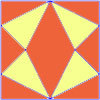Fraction Fascination

Age 7 to 11 Challenge Level:

This problem challenges you to work out what fraction of the whole area of these pictures is taken up by various shapes.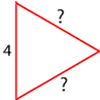Number the Sides

Age 7 to 11 Challenge Level:

The triangles in these sets are similar - can you work out the lengths of the sides which have question marks?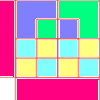Diagrams

Age 7 to 11 Challenge Level:

A group activity using visualisation of squares and triangles.Transformations on a Pegboard

Age 7 to 11 Challenge Level:

How would you move the bands on the pegboard to alter these shapes?Folding

Age 7 to 11 Challenge Level:

What shapes can you make by folding an A4 piece of paper?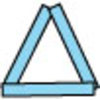Sticks and Triangles

Age 7 to 11 Challenge Level:

Using different numbers of sticks, how many different triangles are you able to make? Can you make any rules about the numbers of sticks that make the most triangles?Egyptian Rope

Age 7 to 11 Challenge Level:

The ancient Egyptians were said to make right-angled triangles using a rope with twelve equal sections divided by knots. What other triangles could you make if you had a rope like this?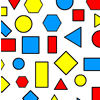Sorting Logic Blocks

Age 5 to 11 Challenge Level:

This interactivity allows you to sort logic blocks by dragging their images.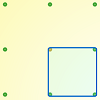Peg and Pin Boards

Age 5 to 11

This article for teachers suggests activities based on pegboards, from pattern generation to finding all possible triangles, for example.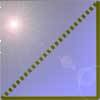Cutting Corners

Age 7 to 11 Challenge Level:

Can you make the most extraordinary, the most amazing, the most unusual patterns/designs from these triangles which are made in a special way?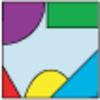Jig Shapes

Age 5 to 11 Challenge Level:

Can you each work out what shape you have part of on your card? What will the rest of it look like?Cutting it Out

Age 5 to 11 Challenge Level:

I cut this square into two different shapes. What can you say about the relationship between them?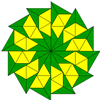Triangle Shapes

Age 5 to 11 Challenge Level:

This practical problem challenges you to create shapes and patterns with two different types of triangle. You could even try overlapping them.Putting Two and Two Together

Age 7 to 11 Challenge Level:

In how many ways can you fit two of these yellow triangles together? Can you predict the number of ways two blue triangles can be fitted together?Shapely Pairs

Age 11 to 14 Challenge Level:

A game in which players take it in turns to turn up two cards. If they can draw a triangle which satisfies both properties they win the pair of cards. And a few challenging questions to follow...Property Chart

Age 11 to 14 Challenge Level:

A game in which players take it in turns to try to draw quadrilaterals (or triangles) with particular properties. Is it possible to fill the game grid?More Transformations on a Pegboard

Age 7 to 11 Challenge Level:

Use the interactivity to find all the different right-angled triangles you can make by just moving one corner of the starting triangle.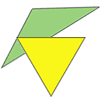Triangle Relations

Age 7 to 11 Challenge Level:

What do these two triangles have in common? How are they related?Triangles All Around

Age 7 to 11 Challenge Level:

Can you find all the different triangles on these peg boards, and find their angles?Nine-pin Triangles

Age 7 to 11 Challenge Level:

How many different triangles can you make on a circular pegboard that has nine pegs?